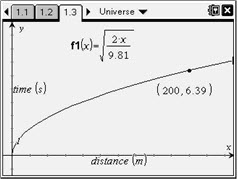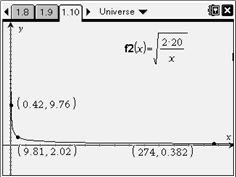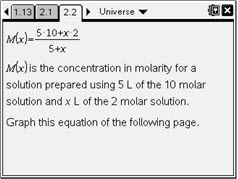# Activities

••• ##### Subject Area

• Math: Precalculus: Rational Functions

• ##### Author9-12

20 Minutes

• ##### Device
•TI-Nspire™ CX/CX II
•TI-Nspire™ CX CAS/CX II CAS
• TI-Nspire™
• TI-Nspire™ CAS
• ##### Software

TI-Nspire™
TI-Nspire™ CAS

2.1

## It's a Radical, Rational Universe!

#### Activity Overview

In this activity, students will explore values and optimization of rational and radical functions in real contexts by graphing and using spreadsheets. Problem 1 involves a radical function relating to acceleration due to gravity. Problem 2 involves a rational function relating to the dilution of a more concentrated solution with a less concentrated solution.

#### Key Steps

•In this problem, students first explore the time required for objects to fall from various distances on Earth. This is done by letting x replace d and 9.81 replace g in the given radical equation. Students are asked questions that may be answered on the handheld related to the graph and function values.

For this exploration, students may use the Point On tool. After a point is placed on the graph of the function, the x-coordinate may be edited and the y‑coordinate will adjust accordingly after pressing enter. If students are unfamiliar with this functionality, it would be helpful for the instructor to guide them through this process. Ask students to predict how the graph will change when x is substituted in place of g and d is replaced with the constant value 20. This is a challenge, but worthy of consideration and discussion.

•Students will then graph the revised function on page 1.10, where x is now gravity, 20 replaces d, and f(x) still represents time. They use the graph and related gravity information for a variety of bodies to identify locations where the time for a body to fall a distance of 20 meters would be the greatest and least. Additional related questions are included.

•Students continue to Problem 2 which is a diluation problem. Dilution problems provide a great opportunity for real world exploration of rational functions. In this problem, students begin with 5 liters of a 10 molar solution of hydrochloric acid and dilute it with a 2 molar solution.